Related Articles
Hierarchical Clustering in R Programming
• Last Updated : 02 Jul, 2020

Hierarchical clustering is an Unsupervised non-linear algorithm in which clusters are created such that they have a hierarchy(or a pre-determined ordering). For example, consider a family of up to three generations. A grandfather and mother have their children that become father and mother of their children. So, they all are grouped together to the same family i.e they form a hierarchy.

Hierarchical clustering is of two types:

• Divisive Hierarchical clustering: It starts at individual leaves and successfully merge clusters together. Its a Bottom up approach.
• Agglomerative Hierarchical clustering: It starts at root and recursively split the clusters. It’s a Top down approach.

#### Theory

In hierarchical clustering, Objects are categorized into a hierarchy similar to tree shaped structure which is used to interpret hierarchical clustering models. The algorithm is as follows:

1. Make each data point in single point cluster that forms N clusters.
2. Take the two closest data points and make them one cluster that forms N-1 clusters.
3. Take the two closest clusters and make them one cluster that forms N-2 clusters.
4. Repeat steps 3 until there is only one cluster.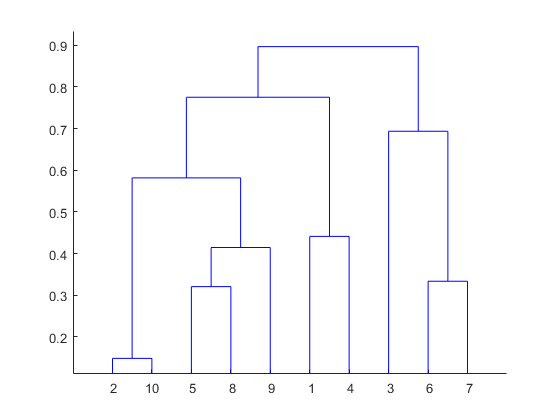Dendrogram is a hierarchy of clusters in which distances are converted into heights. It clustersn units or objects each with p feature into smaller groups. Units in the same cluster are joined by a horizontal line. The leaves at the bottom represent individual units. It provides a visual representation of clusters.

Thumb Rule: Largest vertical distance which doesn’t cut any horizontal line defines the optimal number of clusters.

#### The Dataset

`mtcars`(motor trend car road test) comprises fuel consumption, performance and 10 aspects of automobile design for 32 automobiles. It comes pre installed with dplyr package in R.

 `# Installing the package``install.packages(``"dplyr"``)``   ` `# Loading package``library(dplyr)``   ` `# Summary of dataset in package``head(mtcars)`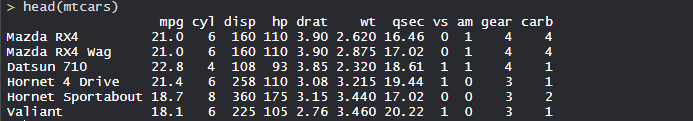#### Performing Hierarchical clustering on Dataset

Using Hierarchical Clustering algorithm on the dataset using `hclust()` which is pre installed in stats package when R is intalled.

 `# Finding distance matrix``distance_mat <``-` `dist(mtcars, method ``=` `'euclidean'``)``distance_mat`` ` `# Fitting Hierarchical clustering Model ``# to training dataset``set``.seed(``240``)  ``# Setting seed``Hierar_cl <``-` `hclust(distance_mat, method ``=` `"average"``)``Hierar_cl`` ` `# Plotting dendrogram``plot(Hierar_cl)`` ` `# Choosing no. of clusters``# Cutting tree by height``abline(h ``=` `110``, col ``=` `"green"``)`` ` `# Cutting tree by no. of clusters``fit <``-` `cutree(Hierar_cl, k ``=` `3` `)``fit`` ` `table(fit)``rect.hclust(Hierar_cl, k ``=` `3``, border ``=` `"green"``)`

#### Output:

• Distance matrix: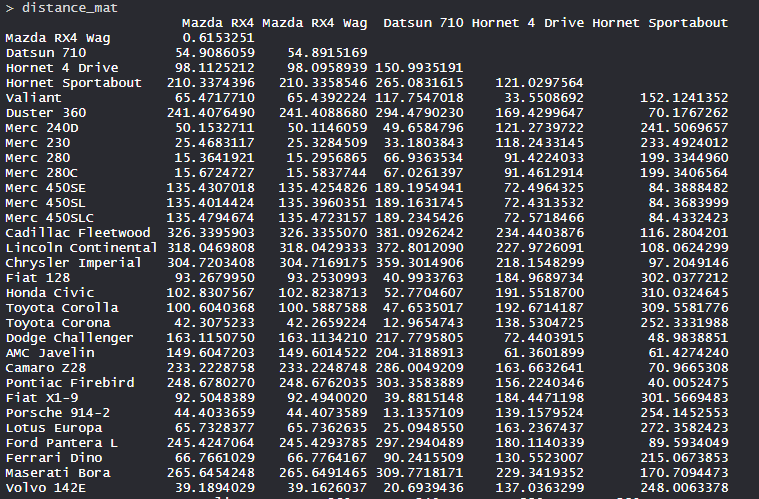The values are shown as per the distance matrix calculation with the method as euclidean.

• Model Hierar_cl: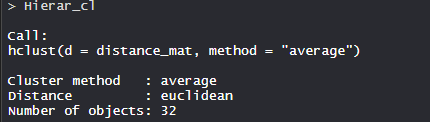In the model, the cluster method is average, distance is euclidean and no. of objects are 32.

• Plot dendrogram: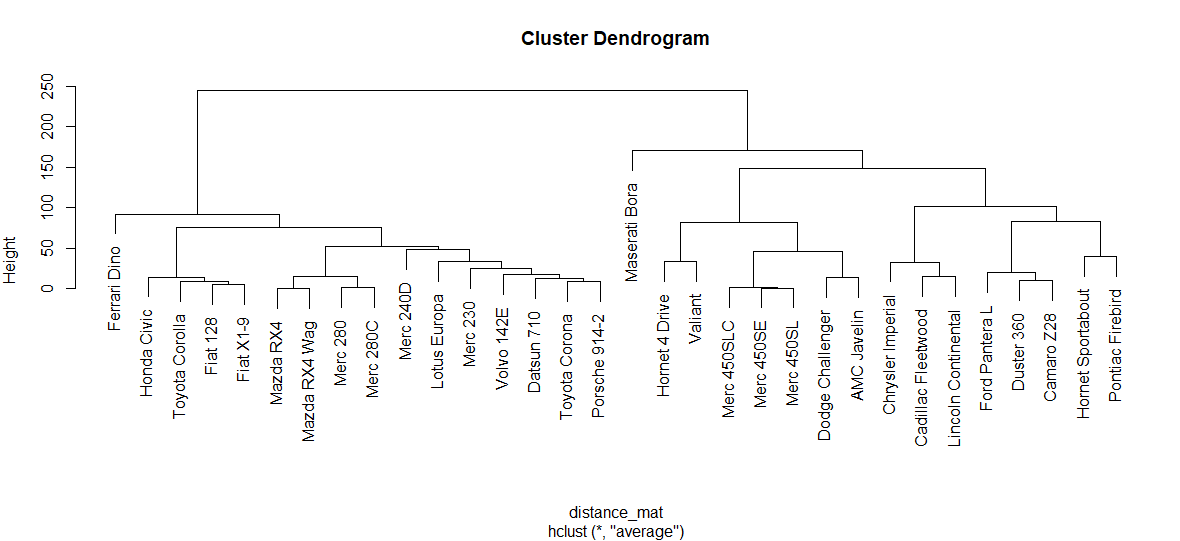The plot dendrogram is shown with x-axis as distance matrix and y-axis as height.

• Cutted tree: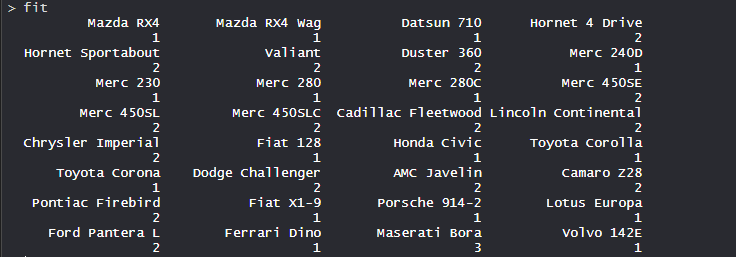So, Tree is cut where k = 3 and each category represents its number of clusters.

• Plotting dendrogram after cutting: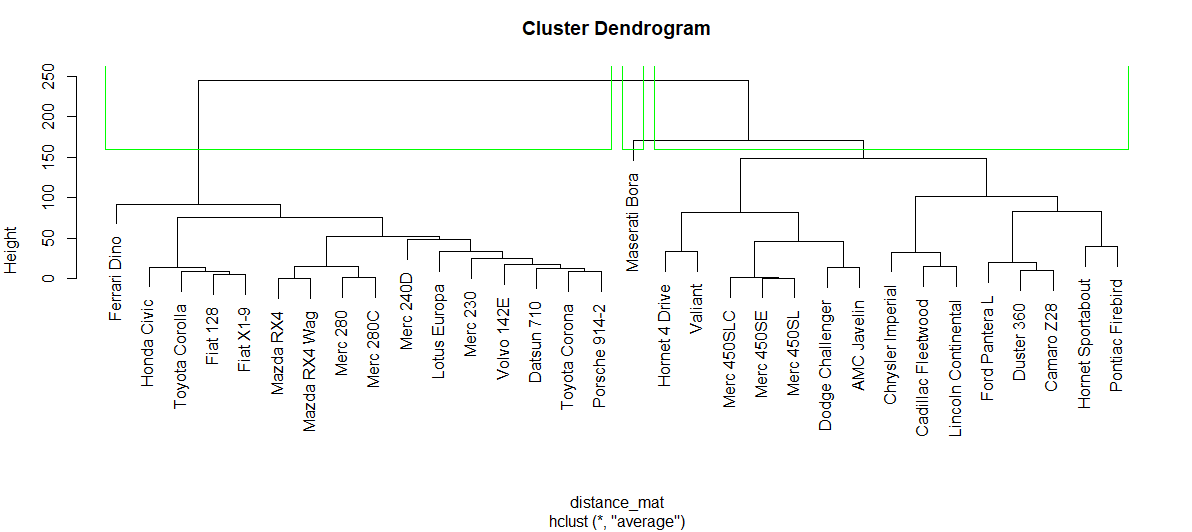The plot denotes dendrogram after being cut. The green lines show the number of clusters as per thumb rule.

So, Hierarchical clustering is widely used in the industry.My Personal Notes arrow_drop_up